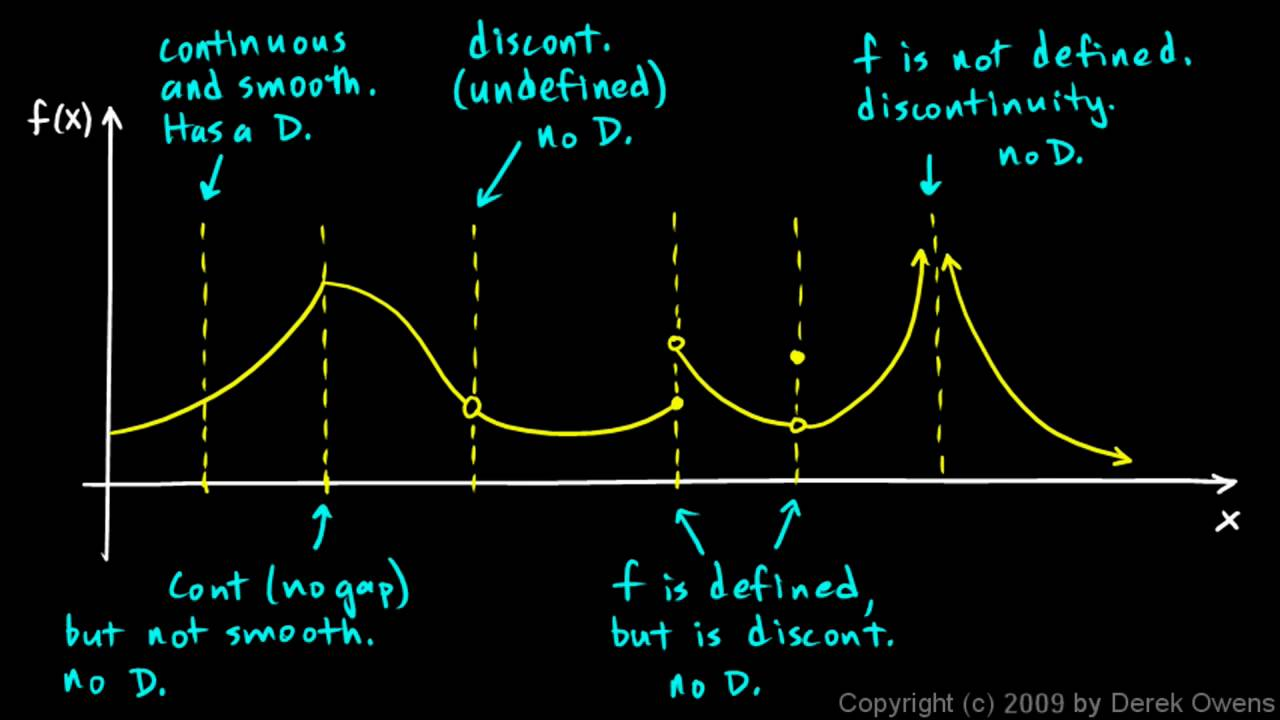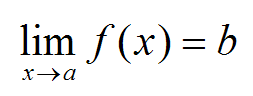# Derivatives made easy 2) Non-differentiable functions

Hi,
so I continue with this tutorial. Today I will cover when function is not differentiable. If you want to read the basics check out the last post, it links to limits and continuity which will be important today.

Just to remind ourselves here is the formula that I explained last time:Basics

It uses limit so logically if the point we are trying to derive is not continous, which means that the limit does not exist there we can not derive. This is not the only thing that can happen to us. Sometimes function is completely continous but from negative side it grows exponentially and from positive side it is linear. Check out the nice picture below:Continuity

From the whole picture the most important point is the second one. It says: “continous (no gap) but not smooth, not differentiable”. To find this out algebraically you need to know the equations of both left and right part of the function.

If you know them you will insert it into the derivation equation that we used last time as f(x). You have to add h of course as it is the difference between your two points. If both of your solutions equal (right and left side) you know that the function IS differentiable.

Dragallur

PS: ask me if you want to expand any part of the post. I have no problem whatsoever with it and it will make me even happy to write some extra parts.

Yes function can be made up of different parts only on intervals. You can even write something like (odd Xs are equal to 2).

# Limits made easy and continuity

Hi,
yesterday I wrote some basics about functions and today I will write about limits again, and when they are defined and when they are not.

Just to remind you, we will use limits on functions. Those functions will usually be plotted on graph so it is easier to work with them. Our first function we work with will be the one below:If you have not seen that yet, filled dot means that the point is inside of the function and circle means that the point is not there. Now when we speak of limit and if it is defined we have to get the same results as we approach from both sides.

What do I mean by this? Limit is basicly just approaching some number very very close. Take for example x=1. At that point in the function above, y equals 5. If we wanted to take the limit it would go as follow:

limx→1   f(x)                           

Now if we think about it before we get the result, we know that you can approach one thing in 1st dimension in two directions. Those are in limits noted as from the negative side (-) and positive side (+).

For the limit I wrote, (limx→1   f(x)) from both sides we will get the normal result as if we wanted to know what the function outputs but sometimes we just can not use the limit because  it does not exist.

Such an example would be for the following limit (still working with the first function):

limx→2   f(x)

In this limit we are trying to approach the number two but from the graph we can see that it is strange at that point. The function jumps so we should check the limit from both sides.

limx→2+   f(x) = 1
limx→2   f(x) = 2

Since the limits do not equal each other, the one before does not exist! We can have so called “one-sided limits” but they are not of much use as far as I know. Now we can actually create amazing definition for continous function!

Continous function is such that does not have any jumps or spaces in it.The function on the left is discontinous. This is because on one point there is space, which means that function is not defined there.. this is problem if you want to be continous.Another function is discontinous even that it is defined on all Xs. The problem lies in what I told you about, if we took the limitx→3 f(x) = undefined
This is because from positive and negative side we will get different result.Again the function is not continous. This time the  limit at 2 exists but it does not equal the value of the function.

limitx→2 f(x) ≠ f(2)This one on is not continous either. This is because if we approach from negative values we will get -∞ but if we approach from positive values we will get +∞. The limits do not equal so the function can not be continous.

From these points we can summarize what function has to have to be continous at one point.

1. Point there is defined, it is not empty circle!
2. Limit on the point exists.
3. The limit equals to the point on the function.

Or also:

1. f(a) is defined
2. limx→ a f(x) exists
3. limx→ a f(x) = f(a)

This is all from me for now, thanks to “analyzemath.com“. I did not need to create all the graphs again thanks to them.

Dragallur

Sadly wordpress is not able to create some normal indexing so I will write it like this. Normally limit is noted as this:Proper notation for limit where x approaching something is in the bottom.

# Limits made easy and heart equation

Hi,
I said that I started to learn limits. Since often I write about the things I just learn I will start this series today with the very basic. (The start is actually so easy you wont even think that it is precalculus or something)

Basicly the context for limits are functions. I have personally never learned functions onBasic idea of function

their own and I do plan to do that but I do not think it is so important right now. You just
have to have this idea that when you have function you will give it some input and it will give out some output (kind of black box).
It is important to say that one input corresponds only to one output. You have already probably seen some graphs so it is good to say that you can plot a function.

The thing with outputs and inputs can be nicely illustrated on one thing: imagine a class of kids and that you measure them.

Carl – 157 cm
Ann – 152 cm
Caroline – 160 cm
… and so on

Now you will plot them on graph next to each other. In big enough class you would probably have more kids with same height.On the left we have three example kids from one class. As is written, Ann has 152 centimeters, Susan 152 too but Jacob has 149,155 and 161 centimeters. Such class would not make a great function! The interesting thing is of course that to one height (152 cm) you can match more people (Ann and Susan), this makes sense. But you can not match one person to more heights! This is the way function works. You could just change names and heights for x and y and you would be there. So if you graph some function it will never be vertical and basicly no two values will ever be above each other, when you on the other hand plot equation you can easily get graph where there are two points above each other:Equation stolen from twitter and modified for better look.

So… what role do limits play here? As you probably already heard, limit tolds us what value will function give us when we give it input really close to some number. If you have function like

f(x)=x^2                  (f(x) is the way functions are noted, you could use other symbols)

You can ask for limit of x approaching to any real number and you would get the same as if you would calculate x to the power of 2. The real usage of limits comes when there is point where you can not get some nice value and also I think that it is defined by limits if function is continous (without jumps) or not.

I will slowly continue in the next post 😉

Dragallur

PS: some of my readers mathematicians (or anybody), if there please point out any mistakes, I am just learning this so it would be great to know my mistakes!

 To make it even clearer I made this extra picture: